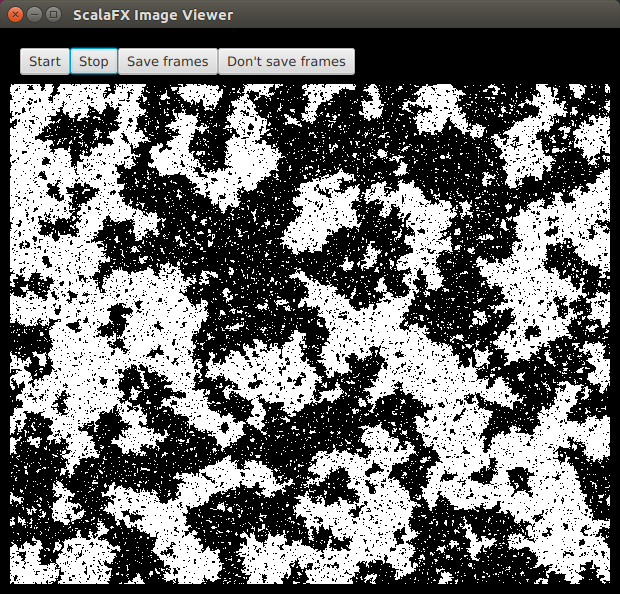# Scala-view: Animate streams of images

## Introduction

In the previous post I discussed how comonads can be useful for structuring certain kinds of scientific and statistical computations. Two of the examples I gave were concerned with the time-evolution of 2-d images. In that post I used Breeze to animate the sequence of computed images. In this post I want to describe an alternative that is better suited to animating an image sequence.

Scala-view is a small Scala library for animating a Stream of Images on-screen in a separate window managed by your window manager. It works with both ScalaFX `Images` (recommended) and Scala Swing/AWT `BufferedImages` (legacy). The stream of images is animated in a window with some simple controls to start and stop the animation, and to turn on and off the saving of image frames to disk (typically for the purpose of turning the image sequence into a movie). An example of what a window might look like is given below.More comprehensive documentation is available from the scala-view github repo, but here I give a quick introduction to the library to outline its capabilities.

## A Scala-view tutorial

This brief tutorial gives a quick introduction to using the Scala-view library for viewing a ScalaFX Image Stream. It assumes only that you have SBT installed, and that you run SBT from an empty directory.

## An SBT REPL

Start by running SBT from an empty or temporary directory to get an SBT prompt:

```\$ sbt
>
```

Now we need to configure SBT to use the Scala-view library, and start a console. From the SBT prompt:

```set libraryDependencies += "com.github.darrenjw" %% "scala-view" % "0.5"
set scalaVersion := "2.12.4"
console
```

The should result in a `scala>` REPL prompt. We can now use Scala and the Scala-view library interactively.

## An example REPL session

You should be able to paste the code snippets below directly into the REPL. You may find `:paste` mode helpful.

We will replicate the heat equation example from the examples-sfx directory, which is loosely based on the example from my blog post on comonads. We will start by defining a simple parallel Image and corresponding comonadic pointed image `PImage` type. If you aren’t familiar with comonads, you may find it helpful to read through that post.

```import scala.collection.parallel.immutable.ParVector
case class Image[T](w: Int, h: Int, data: ParVector[T]) {
def apply(x: Int, y: Int): T = data(x * h + y)
def map[S](f: T => S): Image[S] = Image(w, h, data map f)
def updated(x: Int, y: Int, value: T): Image[T] =
Image(w, h, data.updated(x * h + y, value))
}

case class PImage[T](x: Int, y: Int, image: Image[T]) {
def extract: T = image(x, y)
def map[S](f: T => S): PImage[S] = PImage(x, y, image map f)
def coflatMap[S](f: PImage[T] => S): PImage[S] = PImage(
x, y, Image(image.w, image.h,
(0 until (image.w * image.h)).toVector.par.map(i => {
val xx = i / image.h
val yy = i % image.h
f(PImage(xx, yy, image))
})))
def up: PImage[T] = {
val py = y - 1
val ny = if (py >= 0) py else (py + image.h)
PImage(x, ny, image)
}
def down: PImage[T] = {
val py = y + 1
val ny = if (py < image.h) py else (py - image.h)
PImage(x, ny, image)
}
def left: PImage[T] = {
val px = x - 1
val nx = if (px >= 0) px else (px + image.w)
PImage(nx, y, image)
}
def right: PImage[T] = {
val px = x + 1
val nx = if (px < image.w) px else (px - image.w)
PImage(nx, y, image)
}
}
```

We will need a function to convert this image into a ScalaFX `WritableImage`.

```import scalafx.scene.image.WritableImage
import scalafx.scene.paint._
def toSfxI(im: Image[Double]): WritableImage = {
val wi = new WritableImage(im.w, im.h)
val pw = wi.pixelWriter
(0 until im.w) foreach (i =>
(0 until im.h) foreach (j =>
pw.setColor(i, j, Color.gray(im(i,j)))
))
wi
}
```

We will need a starting image representing the initial condition for the heat equation.

```val w = 600
val h = 500
val pim0 = PImage(0, 0, Image(w, h,
((0 until w*h).toVector map {i: Int => {
val x = i / h
val y = i % h
0.1*math.cos(0.1*math.sqrt((x*x+y*y))) + 0.1 + 0.8*math.random
}}).par
))
```

We can define a kernel associated with the update of a single image pixel based on a single time step of a finite difference solution of the heat equation.

```def kernel(pi: PImage[Double]): Double = (2*pi.extract+
pi.up.extract+pi.down.extract+pi.left.extract+pi.right.extract)/6.0
```

We can now create a `Stream` of `PImage` with

```def pims = Stream.iterate(pim0)(_.coflatMap(kernel))
```

We can turn this into a `Stream[WritableImage]` with

```def sfxis = pims map (im => toSfxI(im.image))
```

Note that we are essentially finished at this point, but so far everything we have done has been purely functional with no side effects. We haven’t even computed our solution to the heat equation. All we have constructed are lazy infinite streams representing the solution of the heat equation.

Finally, we can render our Stream of Images on screen with

```scalaview.SfxImageViewer(sfxis,1e7.toInt)
```

which has a delay of 1e7 nanoseconds (10 milliseconds) between frames.

This should pop up a window on your display containing the initial image. Click on the Start button to animate the solution of the heat equation. See the API docs for SfxImageViewer for additional options. The ScalaFX API docs may also be useful, especially the docs for Image and WritableImage.